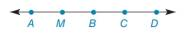Chapter 1.3, Problem 17E### Elementary Geometry for College St...

6th Edition
Daniel C. Alexander + 1 other
ISBN: 9781285195698

#### Solutions

Chapter
Section### Elementary Geometry for College St...

6th Edition
Daniel C. Alexander + 1 other
ISBN: 9781285195698
Textbook Problem
1 views

# In Exercises 9 to 28, use the drawings as needed to answer the following questions.Exercises 14 − 17 Given: A M = 2 x + 1 , M B = 3 x + 2 , and A B = 6 x − 4 Find: x and A B

To determine

To find:

The values of x and AB.

Explanation

Given:

The given figure is,

The given relations are,

AM=2x+1, MB=3x+2, and AB=6x4.

Definition:

If M is point on line segment AB and AMB, then AM+MB=AB.

Calculation:

Consider the given figure,

Figure (1)

Use Segment-Addition Postulate for points A, M and B.

AM+MB=AB(1)

Substitute 2x+1 for AM, 3x+2 for MB and 6x4 for AB in equation (1)

### Still sussing out bartleby?

Check out a sample textbook solution.

See a sample solution

#### The Solution to Your Study Problems

Bartleby provides explanations to thousands of textbook problems written by our experts, many with advanced degrees!

Get Started

#### In Exercises 5-8, find the limit. limx01x(x+x)

Calculus: An Applied Approach (MindTap Course List)

#### Find the value of c such that the point (4, 2) lies on the graph of f(x) = cx2 + 3x 4.

Applied Calculus for the Managerial, Life, and Social Sciences: A Brief Approach

#### Define the validity of measurement and explain why and how it is measured.

Research Methods for the Behavioral Sciences (MindTap Course List)